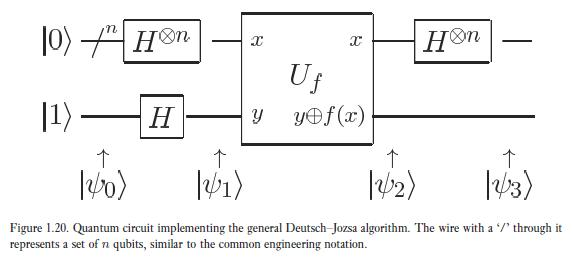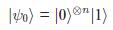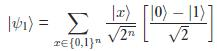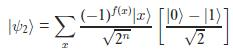### Deutsch-Jozsa Algorithm (to be zero or not)

The problem to which this algorithm suggests solution is that there is an input set of all possible values of n bits, there is a function whose global property is to be constant or balanced which means it takes either all the inputs to a constant value or half of the inputs to a value and other half of the inputs to another value. $$f: \{0,1\}^n\rightarrow \{0,1\}$$. Element number of input set is $$2^n$$. Element number of output set is either 1 or 2. And global propert of $$f$$ will be determined. A classical computer solves this problem with $$\frac{2^n}{2}+1$$ number of queries. What about a quantum computer uses D-J Alg.? It solves this problem in which number of queries?Input state's space is $$2^{n+1}$$ dimensional.After $$\ket{\psi_0}$$, we apply Hadamard gate to $$n+1$$ qubits. We combine $$n$$ qubits with Kronecker product. Now we have the superposition of all the basis of $$2^n$$ dimensional space and the last qubit:Now, we are in the stage of applying the unitary transformation $$U_f$$. n bunch of qubits gidiği gibi çıkıyor, the function $$f(x)$$ is applied onto the last qubit only. $$f(x)$$ is either constant or balanced, in all the situations the last qubit will change according to $$\ket{0\oplus f(x)}-\ket{1\oplus f(x)}$$. Let's say $$f(x)$$ is constant and is equal to $$0$$. Then, the last qubit remains as it is, n bunch of qubits too. Let's say $$f(x)$$ is constant and is equal to $$1$$. Then, the last qubit becomes $$\frac{-\ket{0}+\ket{1}}{\sqrt{2}}=-\frac{\ket{0}-\ket{1}}{\sqrt{2}}$$. n bunch of qubits expression doesn't change. Only, we see either $$+$$ or $$-$$ caused by the last qubit. So general expression in case of constant function:If $$f(x)=0$$ for all the inputs, $$\ket{\psi_2}$$ has $$+$$ sign. If $$f(x)=1$$ for all the inputs, $$\ket{\psi_2}$$ has $$-$$ sign.

$$\ket{\psi_3}=\ket{0}^{\otimes n} (-1)^{f(x)}\frac{\ket{0}-\ket{1}}{\sqrt{2}}$$

First n-qubit is called as registers, last qubit is called as target qubit. Registers constitute the input set in the problem. Last qubit is just like an ancillary qubit.

Let's assume $$n=2$$. If $$f(x)$$ is balanced and:

case III:
• $$f(00)=0$$
• $$f(01)=0$$
• $$f(10)=1$$
• $$f(11)=1$$

It means that if 1.qubit is 0, do nothing to 3.qubit. If 1.qubit is 1, flip 3.qubit. (2.kübit etkisiz eleman.)

$$\ket{\psi_1}=\frac{1}{\sqrt{4}}(\ket{00}+\ket{01}+\ket{10}+\ket{11})\frac{\ket{0}-\ket{1}}{\sqrt{2}}$$

In $$\ket{\psi_3}$$, last qubit will change according to first qubit. So group qubits according to first qubit:

$$\ket{\psi_2}=\frac{1}{\sqrt{4}}\big((\ket{00}+\ket{01})\frac{\ket{0}-\ket{1}}{\sqrt{2}}+(\ket{10}+\ket{11})\frac{\ket{0}-\ket{1}}{\sqrt{2}}\big)$$

If first qubit is 1, flip third qubit:

$$\ket{\psi_2}=\frac{1}{\sqrt{4}}\big((\ket{00}+\ket{01})\frac{\ket{0}-\ket{1}}{\sqrt{2}}+(\ket{10}+\ket{11})\frac{\ket{1}-\ket{0}}{\sqrt{2}}\big)$$

Take $$-$$ sign from last qubit and use it in first and second qubit:

$$\ket{\psi_2}=\frac{1}{\sqrt{4}}\big((\ket{00}+\ket{01})\frac{\ket{0}-\ket{1}}{\sqrt{2}}+(-\ket{10}-\ket{11})\frac{\ket{0}-\ket{1}}{\sqrt{2}}\big)$$

$$\ket{\psi_2}=\frac{\ket{0}-\ket{1}}{\sqrt{2}} \frac{\ket{0}+\ket{1}}{\sqrt{2}} \frac{\ket{0}-\ket{1}}{\sqrt{2}}$$

$$\ket{\psi_3}=\ket{10}\frac{\ket{0}-\ket{1}}{\sqrt{2}}$$

case IV:
• $$f(00)=1$$
• $$f(01)=1$$
• $$f(10)=0$$
• $$f(11)=0$$

It means that if 1.qubit is 0, flip 3.qubit. If 1.qubit is 1, do nothing to 3.qubit.

$$\ket{\psi_3}=-\ket{10}\frac{\ket{0}-\ket{1}}{\sqrt{2}}$$

case V:
• $$f(00)=0$$
• $$f(01)=1$$
• $$f(10)=0$$
• $$f(11)=1$$

It means that if 2.qubit is 0, do nothing to 3.qubit. If 2.qubit is 1, flip 3.qubit.

$$\ket{\psi_3}=\ket{01}\frac{\ket{0}-\ket{1}}{\sqrt{2}}$$

case VI:
• $$f(00)=1$$
• $$f(01)=0$$
• $$f(10)=1$$
• $$f(11)=0$$

It means that if 2.qubit is 0, flip 3.qubit. If 2.qubit is 1, do nothing to 3.qubit.

$$\ket{\psi_3}=-\ket{01}\frac{\ket{0}-\ket{1}}{\sqrt{2}}$$

As a result, after the measurement of registers if $$\ket{00}$$ is obtained, we understand that $$f(x)$$ is a constant function. If other results than $$\ket{00}$$ is obtained, we understand that $$f(x)$$ is a balanced function. In the generalization, $$\ket{00}$$ becomes $$\ket{0}^{\otimes n}$$ and while a classical computer solves this problem with $$\frac{2^n}{2}+1$$ queries, a quantum computer solves this problem with $$n$$ queries.